Courses

# Test: Communication Systems - 2

## 25 Questions MCQ Test Communication Theory | Test: Communication Systems - 2

Description
This mock test of Test: Communication Systems - 2 for Electronics and Communication Engineering (ECE) helps you for every Electronics and Communication Engineering (ECE) entrance exam. This contains 25 Multiple Choice Questions for Electronics and Communication Engineering (ECE) Test: Communication Systems - 2 (mcq) to study with solutions a complete question bank. The solved questions answers in this Test: Communication Systems - 2 quiz give you a good mix of easy questions and tough questions. Electronics and Communication Engineering (ECE) students definitely take this Test: Communication Systems - 2 exercise for a better result in the exam. You can find other Test: Communication Systems - 2 extra questions, long questions & short questions for Electronics and Communication Engineering (ECE) on EduRev as well by searching above.
QUESTION: 1

### For an ideal 3000 Hz channel, S/N ratio is

Solution: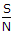= 26000/3000 - 1 = 3.

QUESTION: 2

### Which of the following types of noise assumes greater importance at high frequencies?

Solution:

At high frequencies time period of wave and transit time become comparable.

QUESTION: 3

### In transistor radio receivers the number of IF amplifier stages are

Solution:

Two IF amplifier stages are very commonly used.

QUESTION: 4

To relay outdoor remotely located live programs, TV transmitter use

Solution:

QUESTION: 5

If each short element of signal occupies 20 ms, the speed is

Solution: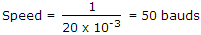.

QUESTION: 6

It is found that in a telephone system 60 subscribers initiate calls during a 30 minute interval and the total duration of all the calls is 100 minutes. The load is

Solution: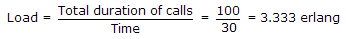.

QUESTION: 7

E and H fields are perpendicular to each other.

Solution:

Radio waves have very high speed equal to that of light but speed of sound waves is very low.

QUESTION: 8

Consider the following:

1. A TV receiver antenna should pick up the TV signal and develop maximum voltage from the available strength of signal
2. It should reject unwanted signals
3. It should not suffer from co-channel interference
4. It should have a very narrowband
The correct statements are
Solution:

The band should not be narrow.

QUESTION: 9

If transmission bandwidth is doubled in FM, SNR is

Solution:

SNR changes in the ratio of square of change in bandwidth.

QUESTION: 10

Directivity means

Solution:

Directivity means maximum directive gain.

QUESTION: 11

If the oscillator output is modulated by audio frequencies upto 10 kHz, the frequency range occupied by the side bands will be

Solution:

L = 50 mH, C = ln F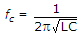.

QUESTION: 12

The power to the portable unit of a cordless telephone is provided by

Solution:

Rechargeable cells feed the portable unit.

QUESTION: 13

In a AM wave the carrier and one of the side bands is suppressed. If m = 0.5, the percentage saving in power is

Solution: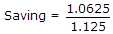.

QUESTION: 14

Computer communication networks have

Solution:

All the three configurations are used.

QUESTION: 15

Assertion (A): A balun is an RF impedance matching transformer.

Reason (R): Sometimes a booster amplifier is used with a TV set.

Solution:

Balun gives a 4 : 1 impedance transformation. In areas where TV reception is poor, booster is used to strengthen a TV signal.

QUESTION: 16

In an FM system, when the AF is 500 Hz and the AF voltage is 2.4 V, the deviation is 4.8 kHz. If the AF voltage is now increased to 7.2 V, the new deviation will be

Solution: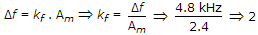Δf' = 2 x 7.2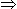14.4 kHz

New frequency donation will be 14.4 kHz.

QUESTION: 17

Modulation is also called detection.

Solution:

Demodulation is called detection.

QUESTION: 18

The maximum range of a transmitter depends on

Solution:

Both power and frequency determine the maximum range.

QUESTION: 19

Consider the following statements about Yagi Uda antenna

1. It is unidirectional
2. Its gain is about 7 dB
3. It is used at VLF
4. The optimum separation is 0.11
Which of the above are correct?
Solution:

This antenna is for UHF and VHF bands.

QUESTION: 20

In a two stage cascade amplifier, each stage has a gain of 10 dB and noise figure of 10 dB. The overall noise factor is

Solution: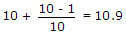.

QUESTION: 21

Fields are said to be circularly polarised if their magnitudes are

Solution:

Magnitudes should be equal and phase angle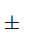90º.

QUESTION: 22

In a CD player the speed of CD is

Solution:

Since the circumference of outer tracks is more than that of tracks near the centre, the speed of disc is varied from 200 rpm to 500 rpm.

QUESTION: 23

Three analog signals, having bandwidths 1200 Hz, 600 Hz, 600 Hz are sampled at their Nyquist rates, encoded with 12 bit words, and time division multiplexed. The bit rate for the multiplexed signal is

Solution:

The total message bandwidth = 1200 Hz + 600 Hz + 600 Hz = 2400 Hz

Sampling frequency = 4800 samples/sec.

Hence, Bit rate = 4800 x 1257.6 kbps.

QUESTION: 24

In the output of a DM speech encoder, the consecutive pulses are of opposite polarity during time interval t1 < t < t2. This indicates that during this interval

Solution:

In delta modulator, when consecutive pulses of polarity are opposite then signal is constant.

QUESTION: 25

A fascimile reproduction has a specification of 200 lines per frame, progressive scanning and 5 frames per second. The time to scan one line is

Solution: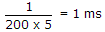.